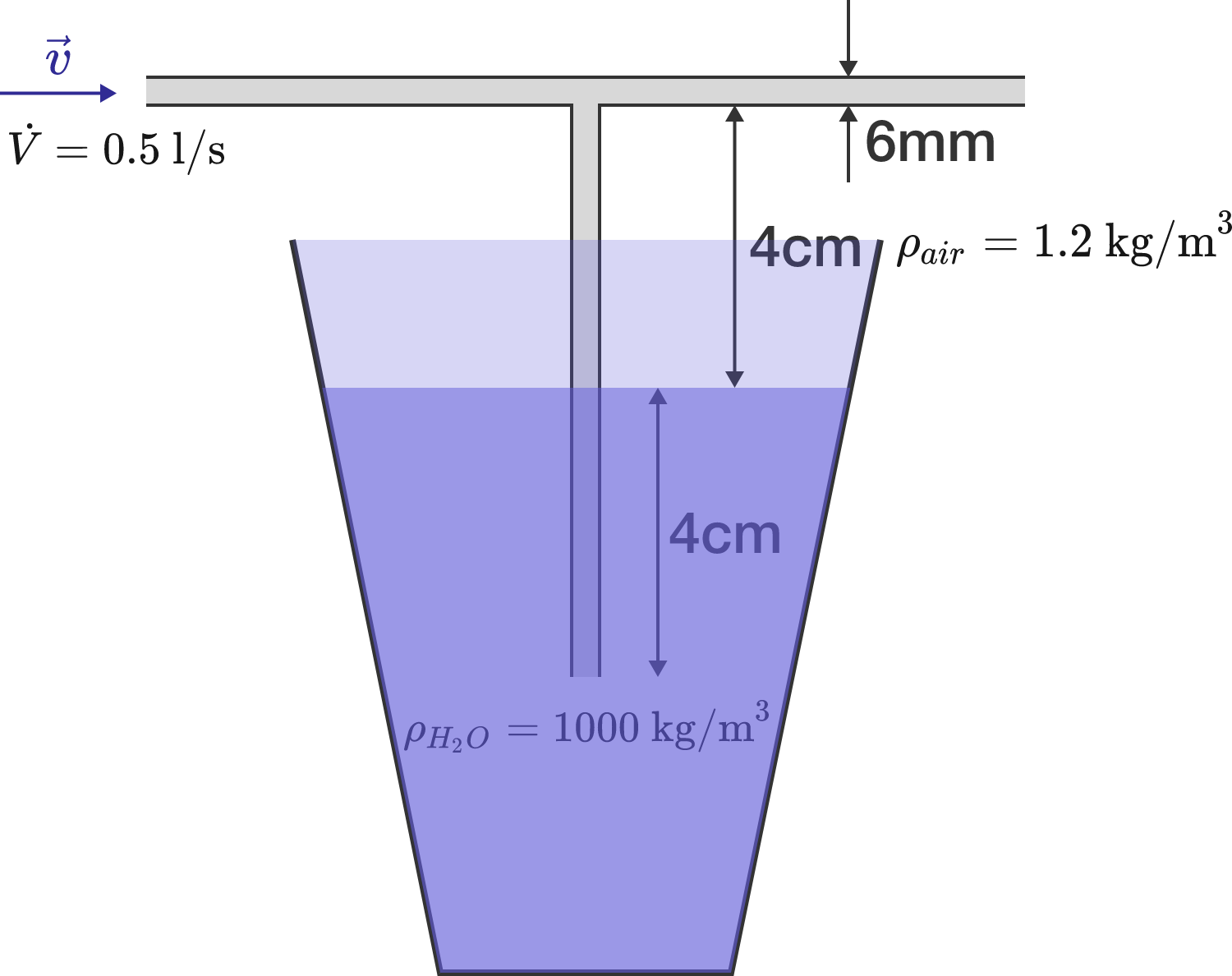# Blowing air through a strawAs shown in the diagram, a T-shaped straw is dipped into a glass of water. If you blow air through the opening on the left, leaving the opening on the right open, what will happen?

Details and Assumptions:

• The incoming air flow is $\dot V = 0.5\, \text{l/s}.$
• The straw with diameter $6 \,\text{mm}$ has a length of $8 \,\text{cm},$ half of which is immersed in the water.
• The densities of air and water are $\rho = 1.2 \,\text{kg}/\text{m}^3$ and $1000 \,\text{kg}/\text{m}^3$, respectively.
• The viscosity of air is neglected so that there is no pressure drop along the pipes.
×

Problem Loading...

Note Loading...

Set Loading...